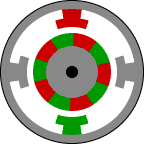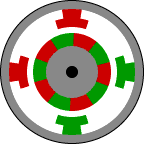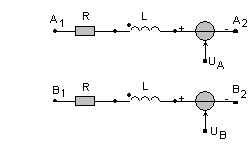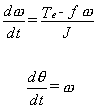• Introduction
• What is in this manual
• What is Caspoc
• User interface
• Introduction
• Starting
• Simulation
• Editing
• Viewing and printing
• Getting Started
• Basic editing
• Simulation in the time domain
• Basic User Interface Topics
• Editing
• Simulation
• Viewing
• Library
• Reports
• Project management
• Circuit and Block Diagram Components
• Introduction
• Cscript and user defined functions
• Component parameters
• Modeling Topics
• Introduction
• Power Electronics
• Semiconductors
• Electrical Machines
• Electrical drives
• Power Systems
• Mechanical Systems
• Thermal Systems
• Magnetic Circuits
• Green Energy
• Coupling to FEM
• Experimenter
• Analog hardware description language
• Embedded C code Export
• Coupling to Spice
• Small Signal Analysis
• Matlab coupling
• Tips and tricks
• Appendices

## Stepper Motors

Full Step Stepper MotorClick to Close this View This animation demonstrates the principle for a stepper motor using full step commutation. The rotor of a permanent magnet stepper motor consists of permanent magnets and the stator has two pairs of windings. Just as the rotor aligns with one of the stator poles, the second phase is energized. The two phases alternate on and off and also reverse polarity. There are four steps. One phase lags the other phase by one step. This is equivalent to one forth of an electrical cycle or 90�.

This stepper motor is very simplified. The rotor of a real stepper motor usually has many poles. The animation has only ten poles, however a real stepper motor might have a hundred. These are formed using a single magnet mounted inline with the rotor axis and two pole pieces with many teeth. The teeth are staggered to produce many poles. The stator poles of a real stepper motor also has many teeth. The teeth are arranged so that the two phases are still 90� out of phase.

This stepper motor uses permanent magnets. Some stepper motors do not have magnets and instead use the basic principles of a switched reluctance motor. The stator is similar but the rotor is composed of a iron laminates.

Half Step Stepper MotorClick to Close this View This animation shows the stepping pattern for a half-step stepper motor. The commutation sequence for a half-step stepper motor has eight steps instead of four. The main difference is that the second phase is turned on before the first phase is turned off. Thus, sometimes both phases are energized at the same time. During the half-steps the rotor is held in between the two full-step positions.

A half-step motor has twice the resolution of a full step motor. It is very popular for this reason.

The stepper motor is available in the library and is build from discrete block diagram and circuit components.

The library blocks for the stepper motors have electrical and mechanical connections. The electrical part of the stepper is modeled by a series connection of the stator inductance, resistance and emf.

An equivalent circuit models the electrical part of the stepper motor, as shown below:The emf induced in the stator windings is modeled by the voltage sources uA and uB:

uA=Ke*sin((p/2)θ)
uB=Ke*cos((p/2)θ)

where Ke is the motor torque constant and p is the number of pole pairs.

The torque produced by the stepper motor is given by:

Te=Ke*(p/2)*(iAsin((p/2)θ)+iBcos((p/2)θ))

The mechanical shaft is modeled by a rigid shaft model. The inertia J [kgm2]of the shaft can be modeled in most of the stepper motor library models. Most machine models have internal parameters for modeling the inertia and friction of the shaft.

The load of the stepper motor is modeled at the component level. Only the friction and the inertia of the load are modeled given by:The stepper motor can either run without load or can be connected to a mechanical rotational model. Use the models from components/circuit/rotational and from the various mechanical libraries for modeling the mechanical model.